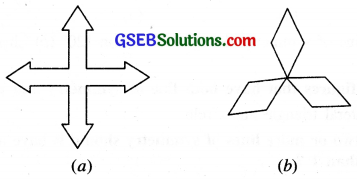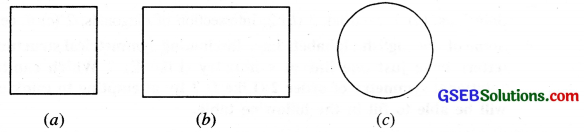# GSEB Class 7 Maths Notes Chapter 14 Symmetry

This GSEB Class 7 Maths Notes Chapter 14 Symmetry covers all the important topics and concepts as mentioned in the chapter.

## Symmetry Class 7 GSEB Notes

Symmetry is an important geometrical concept that it is used almost in every field of daily life. Artists, professionals, designers car manufacturers, architects and many others make use of the idea of symmetry. The beehives, the flowers, the tree-leaves, religious symbols, fish, and birds-everywhere you find symmetrical designs.

• Asymmetrical Figures: The objects or figures that do not have any line of symmetry are called asymmetrical figures.
• Line of Symmetry: A figure has line symmetry, if there is a line about which the figure may be folded so that the two parts of the figure will coincide.
• Mirror reflection: Mirror reflection leads to symmetry, under which the left right orientation have to be taken care of.
• Regular Polygon: A polygon is said to be regular if all its sides are of equal length and all its angles are of equal measure.
Each regular polygon has as many line of symmetry as it has number of sides.

Some Regular Polygons with lines of symmetry

 Name of Regular polygons and their feature Figure with lines of symmetry No. of lines of Symmetry 1. An Equilateral triangle is regular. Each of its sides has same length and each of its angles measures 600.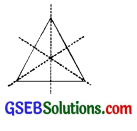3 2. A square is also regular because its sides are all of equal length and each of its angles is a right angle (i.e. 900). Its diagonals are perpendicular bisectors of each other.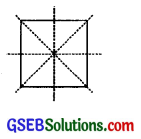4 3. A regular pentagon has all its sides equal and hence each of its angles measures 108°.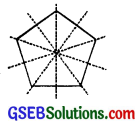5

Rotational Symmetry
In our daily life we see objects that rotate. Rotation is the circular movement of an object about a point. Rotation can be clockwise or anticlockwise.
Figure which on rotation through an angle, about a point, look the same as originals, are said to have rotational Symmetry.Centre of rotation:
The fixed point about which an object rotated is called centre of rotation. For example, the centre of rotation for the hands of clock is the point where all the three hands are joined.

Angle of Rotation: The smallest angle through which an object (or a figure) rotates about a fixed point (Centre of rotation) so that it looks the same is called angle of rotation.

Rotation are of two types
Rotation moving like the hands of a clock is called Clockwise rotation; otherwise it is said to be anticlockwise.

Order of rotational symmetry. If A° is the smallest angle through which a figure can be rotated and skill looks the same, then it has a rotational symmetry of order = $$\frac{360}{\mathrm{~A}^{\circ}}$$

For a figure having a rotational symmetry, A0 must be less than or equal to 180°.

Line symmetry and rotational symmetry. Some figures have only line symmetry, some have rotational symmetry and some have both line symmetry and rotational symmetry for example:

• An isosceles triangle has a line symmetry but not rotational symmetry
• A parallelogram has rotational symmetry but no line symmetry.
• A square has both line symmetry as well as rotational symmetry’.
• A circle is the most perfect symmetrical figure having infinite number of lines of symmetry and it can be rotated about its centre at any angle and still appears the same.

Example: 1
In the following figures, find the number of symmetry and angle of rotation for rotational symmetry.